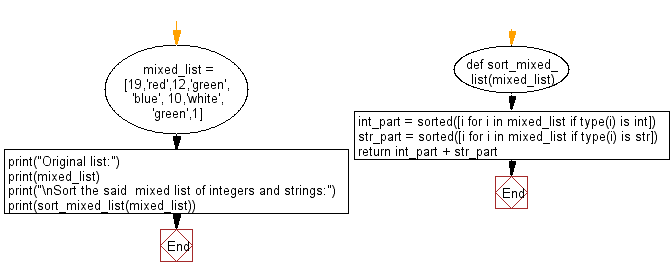﻿ Python: Sort a given mixed list of integers and strings - w3resource# Python: Sort a given mixed list of integers and strings

## Python List: Exercise - 138 with Solution

Write a Python program to sort a given mixed list of integers and strings. Numbers must be sorted before strings.

Sample Solution:

Python Code:

``````def sort_mixed_list(mixed_list):
int_part = sorted([i for i in mixed_list if type(i) is int])
str_part = sorted([i for i in mixed_list if type(i) is str])
return int_part + str_part
mixed_list = [19,'red',12,'green','blue', 10,'white','green',1]
print("Original list:")
print(mixed_list)
print("\nSort the said  mixed list of integers and strings:")
print(sort_mixed_list(mixed_list))
```
```

Sample Output:

```Original list:
[19, 'red', 12, 'green', 'blue', 10, 'white', 'green', 1]

Sort the said  mixed list of integers and strings:
[1, 10, 12, 19, 'blue', 'green', 'green', 'red', 'white']
```

Flowchart:## Visualize Python code execution:

The following tool visualize what the computer is doing step-by-step as it executes the said program:

Python Code Editor:

Have another way to solve this solution? Contribute your code (and comments) through Disqus.

What is the difficulty level of this exercise?

Test your Python skills with w3resource's quiz

﻿

## Python: Tips of the Day

```print(2_000_000)
```2000000